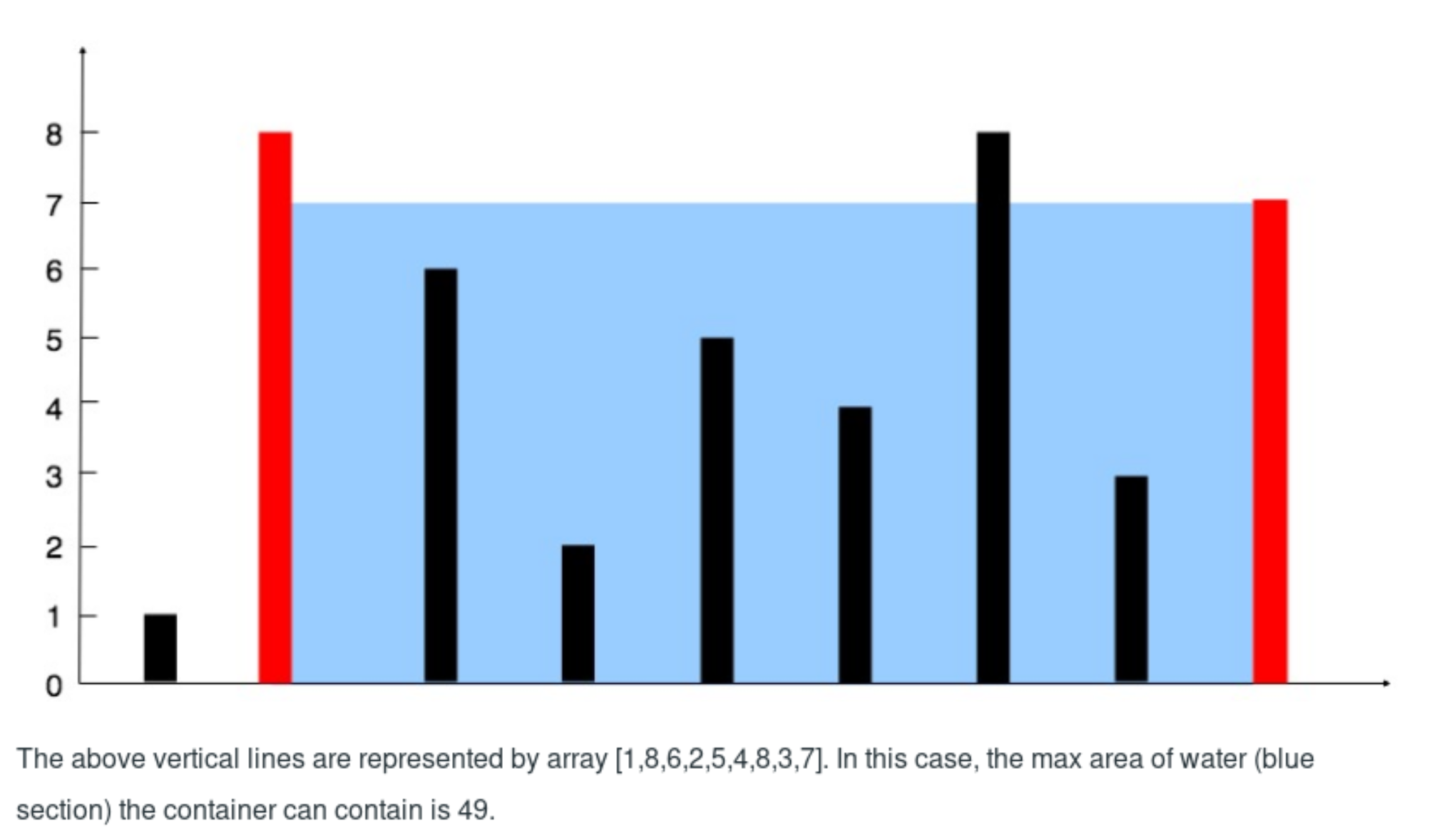0%

## Problem

Given n non-negative integers a1, a2, …, an , where each represents a point at coordinate (i, ai). n vertical lines are drawn such that the two endpoints of line i is at (i, ai) and (i, 0). Find two lines, which together with x-axis forms a container, such that the container contains the most water.

Note: You may not slant the container and n is at least 2.### Examples:

Input: [1,8,6,2,5,4,8,3,7]
Output: 49

## Solutions

• 第一种方法就是暴力法，遍历每种情况，是$$O(n^2)$$复杂度，找到这里面的最大容量的情况，尝试了会超时，所以只能尽量优化到$$O(n)$$，于是有了下一种方法
• 第二种方法是用两个指针，初始情况是一个指向最开头，一个指向结尾，此时的宽度是最大的，然后比较当前两个指针所在位置的高度，低的一方向里面移动，移动会导致宽度减小，所以只能高度低的一边向内移，可能会有更高的。时间复杂度是$$O(n)$$

## 总结

• 这道题思维上很简单，就是找到最大面积的两个值，但是如果简单的暴力法，中等难度的题目很明显没这么无脑，所以直接就超时了
• 之前做过的题目里也用到过两个指针遍历的方法，这样的$$O(n)$$复杂度还是很快的